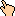## 1997-05 Solution

There are two solutions - the first is my paraphrasing of the answer originally given by Marilyn:
We know he can run just exactly 3/10th of the way forward and get to that end of the bridge at the same time as the train. So, assume he runs away from the train. Then he'll get 3/10th of the way away from his original starting point, or 4/10th of the way across when the train hits the far end of the bridge.

That the train can cover the length of the bridge in the same time as he can cover 4/10th of the bridge means his speed is 4/10th that of the train. (To quickly prove this to yourself, assume that the train and the man started at one end of the bridge together, as racers. If, when the trian clears the bridge at the same time the man clears x percentage of the bridge then the man must run x percentage of the speed of the train). 4/10th of 60mph is 24mph.

and the second solution is the way I solved it:
Let's set some variables:
• `L` is the Land distance from the train to the near end of the bridge.
• `B` is the width of the Bridge
• `S` is the Speed of the man
We know two things - the time it takes for the man to run forward is the same time it takes the train to get to the bridge. Velocity = Distance/Time, so Time = Distance/Velocity, giving us our first expression if the man runs forward:
• `L/60 = .3B/S`
We also know if the man ran away from the train, the train would have to cover more distance and they'd meet at the near side of the bridge. This gives us:
• `(L+B)/60 = .7B/S`
Now we have three unknowns and two equations. But watch algebraic magic: From the first equation we know what `L/60` is. So let's replace that in the second expression:
• `(L+B)/60 = L/60 + B/60 = .3B/S + B/60 = .7B/S`
Which when we multiply by `1/B` (assuming `B != 0`) gives us:
• `.3/S + 1/60 = .7/S`
One equation and one unknown! Pretty cool, huh? Solving for S here, we multiply both sides by `60 S` to get:
• `18 + S = 42`
which yields the solution that `S = 24mph`

WWW Maven: Dan Garcia (ddgarcia@cs.berkeley.edu)Send me feedback